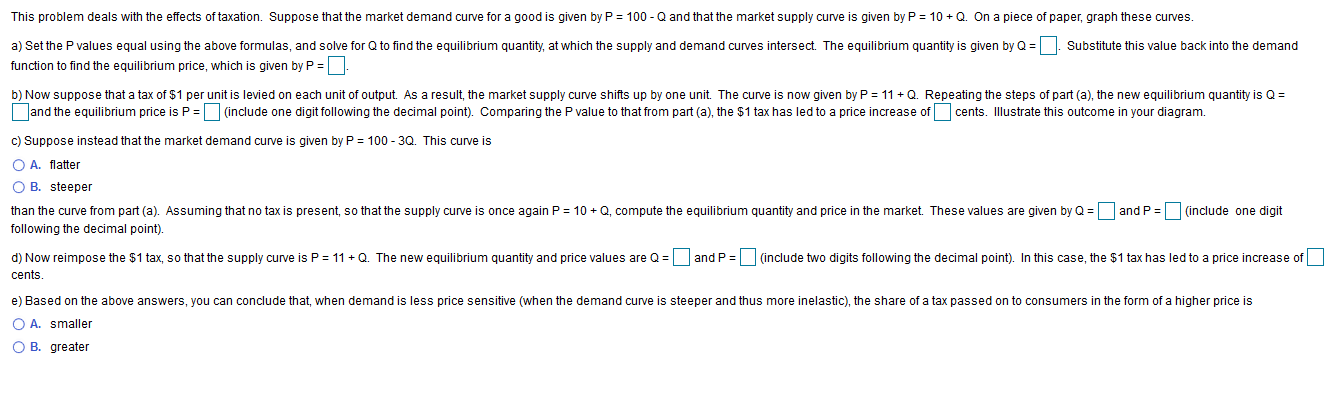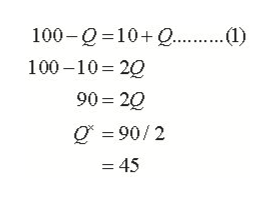# This problem deals with the effects of taxation. Suppose that the market demand curve for a good is given by P100 Q and that the market supply curve is given by P10QOn a piece of paper, graph these curves.a) Set the P values equal using the above formulas, and solve for Q to find the equilibrium quantity, at which the supply and demand curves intersect. The equilibrium quantity is given by QSubstitute this value back into the demandfunction to find the equilibrium price, which is given by P .b) Now suppose that a tax of \$1 per unit is levied on each unit of output. As a result, the market supply curve shifts up by one unit. The curve is now given by P = 11 QRepeating the steps of part (a), the new equilibrium quantity is Qand the equilibrium price is P (include one digit following the decimal point). Comparing the P value to that from part (a), the \$1 tax has led to a price increase ofcents. Illustrate this outcome in your diagram.c) Suppose instead that the market demand curve is given by P = 100 - 3Q. This curve isO A. flatterO B. steeperthan the curve from part (a). Assuming that no tax is present, so that the supply curve is once again P 10 Q, compute the equilibrium quantity and price in the market. These values are given by Qand P (include one digitfollowing the decimal point).d) Now reimpose the \$1 tax, so that the supply curve is P(include two digits following the decimal point). In this case, the \$1 tax has led to a price increase ofand P11 Q. The new equilibrium quantity and price values are Qcents.e) Based on the above answers, you can conclude that, when demand is less price sensitive (when the demand curve is steeper and thus more inelastic), the share of a tax passed on to consumers in the form of a higher price isO A. smallerO B. greater

Question
80 views

Part a, b, chelp_outlineImage TranscriptioncloseThis problem deals with the effects of taxation. Suppose that the market demand curve for a good is given by P 100 Q and that the market supply curve is given by P 10Q On a piece of paper, graph these curves. a) Set the P values equal using the above formulas, and solve for Q to find the equilibrium quantity, at which the supply and demand curves intersect. The equilibrium quantity is given by Q Substitute this value back into the demand function to find the equilibrium price, which is given by P . b) Now suppose that a tax of \$1 per unit is levied on each unit of output. As a result, the market supply curve shifts up by one unit. The curve is now given by P = 11 Q Repeating the steps of part (a), the new equilibrium quantity is Q and the equilibrium price is P (include one digit following the decimal point). Comparing the P value to that from part (a), the \$1 tax has led to a price increase of cents. Illustrate this outcome in your diagram. c) Suppose instead that the market demand curve is given by P = 100 - 3Q. This curve is O A. flatter O B. steeper than the curve from part (a). Assuming that no tax is present, so that the supply curve is once again P 10 Q, compute the equilibrium quantity and price in the market. These values are given by Q and P (include one digit following the decimal point). d) Now reimpose the \$1 tax, so that the supply curve is P (include two digits following the decimal point). In this case, the \$1 tax has led to a price increase of and P 11 Q. The new equilibrium quantity and price values are Q cents. e) Based on the above answers, you can conclude that, when demand is less price sensitive (when the demand curve is steeper and thus more inelastic), the share of a tax passed on to consumers in the form of a higher price is O A. smaller O B. greater fullscreen
check_circle

Step 1

Hi, since you have posted a question with multiple sub parts, we will be answering only first three parts. If you want other sub parts to be answered, please post the question again and mention the specific subpart.

Step 2

Since in the problem we are given that Demand curve is given as P=100-Q and the Supply curve is given as P=10+Q, we can calculate the equilibrium quantity and equilibrium price by equating the demand and supply given, since in equilibrium, Demand = Supply.

Thus, we have, in equilibrium the equation (1) as:

Therefore, the equilibrium quantity is Q*=45.help_outlineImage Transcriptionclose100-Q =10+ Q . (1) 100 –10= 20 90 = 20 O = 90/2 = 45 fullscreen
Step 3

Now, using the value of Q*=45 and putting the value in Demand equation, P=100-Q,

...

### Want to see the full answer?

See Solution

#### Want to see this answer and more?

Solutions are written by subject experts who are available 24/7. Questions are typically answered within 1 hour.*

See Solution
*Response times may vary by subject and question.
Tagged in